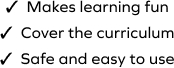# Complete the Decimal Multiplication Pattern

SplashLearn - The complete PreK - Grade 5 Math & ELA Learning Program Built for Your Child
Home > Complete the Decimal Multiplication Pattern
The game challenges young mathematicians to hone their math skills by solving a set of problems on decimal multiplication to find the answer. Students multiply decimals by different powers of 10 and observe the pattern in the products. Students will drag and drop the products at the correct places to solve the problems. Help your fifth grader become proficient in decimal with this game.